Search by Topic

Filter by: Content type:
Age range:
Challenge level:

There are 25 resultsBirthday Sharing

Age 5 to 7 Challenge Level:

It's Sahila's birthday and she is having a party. How could you answer these questions using a picture, with things, with numbers or symbols?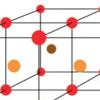Finding 3D Stacks

Age 7 to 11 Challenge Level:

Can you find a way of counting the spheres in these arrangements?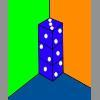Dice in a Corner

Age 7 to 11 Challenge Level:

How could you arrange at least two dice in a stack so that the total of the visible spots is 18?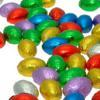Let Us Divide!

Age 7 to 11 Challenge Level:

Look at different ways of dividing things. What do they mean? How might you show them in a picture, with things, with numbers and symbols?Olympic Turns

Age 7 to 11 Challenge Level:

This task looks at the different turns involved in different Olympic sports as a way of exploring the mathematics of turns and angles.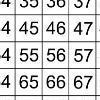That Number Square!

Age 5 to 11 Challenge Level:

Exploring the structure of a number square: how quickly can you put the number tiles in the right place on the grid?Different Sizes

Age 5 to 11 Challenge Level:

A simple visual exploration into halving and doubling.Going for Gold

Age 7 to 11 Challenge Level:

Looking at the 2012 Olympic Medal table, can you see how the data is organised? Could the results be presented differently to give another nation the top place?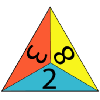One Big Triangle

Age 5 to 7 Challenge Level:

Make one big triangle so the numbers that touch on the small triangles add to 10.Polar Bearings

Age 16 to 18 Challenge Level:

What on earth are polar coordinates, and why would you want to use them?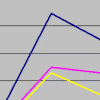Charting More Success

Age 11 to 16 Challenge Level:

Can you make sense of the charts and diagrams that are created and used by sports competitors, trainers and statisticians?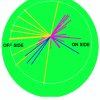Charting Success

Age 11 to 16 Challenge Level:

Can you make sense of the charts and diagrams that are created and used by sports competitors, trainers and statisticians?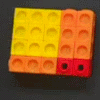Age 14 to 16 Challenge Level:

Can you find out what is special about the dimensions of rectangles you can make with squares, sticks and units?3D Stacks

Age 7 to 14 Challenge Level:

Can you find a way of representing these arrangements of balls?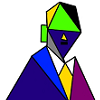Painting by Functions

Age 16 to 18 Challenge Level:

Use functions to create minimalist versions of works of art.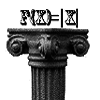Maths Shop Window

Age 16 to 18 Challenge Level:

Make a functional window display which will both satisfy the manager and make sense to the shoppers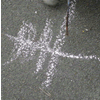Children's Mathematical Graphics: Understanding the Key Concept

Age 5 to 7

In this article for teachers, Elizabeth Carruthers and Maulfry Worthington explore the differences between 'recording mathematics' and 'representing mathematical thinking'.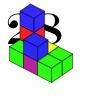28 and It's Upward and Onward

Age 7 to 11 Challenge Level:

Can you find ways of joining cubes together so that 28 faces are visible?The Development of Algebra - 2

Age 11 to 18

This is the second article in a two part series on the history of Algebra from about 2000 BCE to about 1000 CE.What Is the Question?

Age 11 to 16 Challenge Level:

These pictures and answers leave the viewer with the problem "What is the Question". Can you give the question and how the answer follows?Building Gnomons

Age 14 to 16 Challenge Level:

Build gnomons that are related to the Fibonacci sequence and try to explain why this is possible.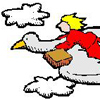A Flying Holiday

Age 7 to 11 Short Challenge Level:

Follow the journey taken by this bird and let us know for how long and in what direction it must fly to return to its starting point.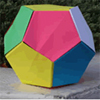Dodecamagic

Age 7 to 11 Challenge Level:

Here you see the front and back views of a dodecahedron. Each vertex has been numbered so that the numbers around each pentagonal face add up to 65. Can you find all the missing numbers?Gnomon Dimensions

Age 14 to 16 Challenge Level:

These gnomons appear to have more than a passing connection with the Fibonacci sequence. This problem ask you to investigate some of these connections.Catrina's Cards

Age 5 to 7 Challenge Level:

How would you find out how many football cards Catrina has collected?